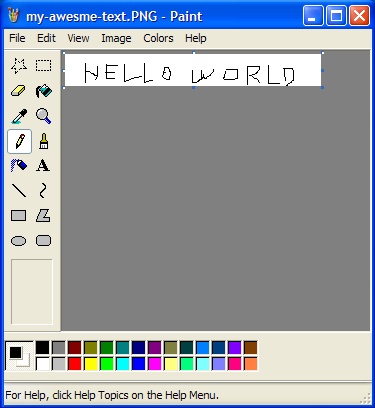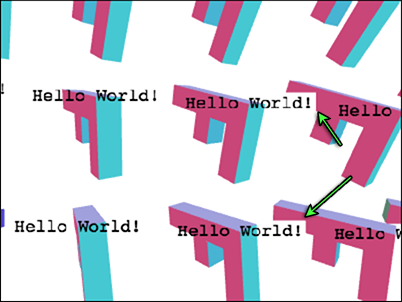# WebGLFundamentals.org

Fix, Fork, Contribute

# WebGL 文字 - 使用纹理``````var textCtx = document.createElement("canvas").getContext("2d");

// 将文字放在画布中间
function makeTextCanvas(text, width, height) {
textCtx.canvas.width  = width;
textCtx.canvas.height = height;
textCtx.font = "20px monospace";
textCtx.textAlign = "center";
textCtx.textBaseline = "middle";
textCtx.fillStyle = "black";
textCtx.clearRect(0, 0, textCtx.canvas.width, textCtx.canvas.height);
textCtx.fillText(text, width / 2, height / 2);
return textCtx.canvas;
}
``````

``````// 创建 'F' 的数据
var fBufferInfo = primitives.create3DFBufferInfo(gl);
// 创建一个单位矩形供文字使用
var textBufferInfo = primitives.createPlaneBufferInfo(gl, 1, 1, 1, 1, m4.xRotation(Math.PI / 2));
``````

``````// 设置着色程序
``````

``````// 创建文字纹理
var textCanvas = makeTextCanvas("Hello!", 100, 26);
var textWidth  = textCanvas.width;
var textHeight = textCanvas.height;
var textTex = gl.createTexture();
gl.bindTexture(gl.TEXTURE_2D, textTex);
gl.texImage2D(gl.TEXTURE_2D, 0, gl.RGBA, gl.RGBA, gl.UNSIGNED_BYTE, textCanvas);
// 确保即使不是 2 的整数次幂也能渲染
gl.texParameteri(gl.TEXTURE_2D, gl.TEXTURE_MIN_FILTER, gl.LINEAR);
gl.texParameteri(gl.TEXTURE_2D, gl.TEXTURE_WRAP_S, gl.CLAMP_TO_EDGE);
gl.texParameteri(gl.TEXTURE_2D, gl.TEXTURE_WRAP_T, gl.CLAMP_TO_EDGE);
``````

``````var fUniforms = {
u_matrix: m4.identity(),
};

var textUniforms = {
u_matrix: m4.identity(),
u_texture: textTex,
};
``````

``````var fViewMatrix = m4.translate(viewMatrix,
fViewMatrix = m4.xRotate(fViewMatrix, rotation);
fViewMatrix = m4.yRotate(fViewMatrix, rotation + yy * xx * 0.2);
fViewMatrix = m4.zRotate(fViewMatrix, rotation + now + (yy * 3 + xx) * 0.1);
fViewMatrix = m4.scale(fViewMatrix, scale, scale, scale);
fViewMatrix = m4.translate(fViewMatrix, -50, -75, 0);
``````

``````gl.useProgram(fProgramInfo.program);

webglUtils.setBuffersAndAttributes(gl, fProgramInfo, fBufferInfo);

fUniforms.u_matrix = m4.multiply(projectionMatrix, fViewMatrix);

webglUtils.setUniforms(fProgramInfo, fUniforms);

// 绘制几何体
gl.drawElements(gl.TRIANGLES, fBufferInfo.numElements, gl.UNSIGNED_SHORT, 0);
``````

``````// 只使用 'F' 视图矩阵的位置
var textMatrix = m4.translate(projectionMatrix,
fViewMatrix, fViewMatrix, fViewMatrix);
// 缩放单位矩形到所需大小
textMatrix = m4.scale(textMatrix, textWidth, textHeight, 1);
``````

``````// 绘制文字设置
gl.useProgram(textProgramInfo.program);

webglUtils.setBuffersAndAttributes(gl, textProgramInfo, textBufferInfo);

m4.copy(textMatrix, textUniforms.u_matrix);
webglUtils.setUniforms(textProgramInfo, textUniforms);

// 绘制文字
gl.drawElements(gl.TRIANGLES, textBufferInfo.numElements, gl.UNSIGNED_SHORT, 0);
``````

``````gl.enable(gl.BLEND);
gl.blendFunc(gl.SRC_ALPHA, gl.ONE_MINUS_SRC_ALPHA);
``````

``````result = dest * (1 - src_alpha) + src * src_alpha
``````

``````src = [1, 0, 0, 1]
dst = [0, 1, 0, 1]
src_alpha = src  // 这是 1
result = dst * (1 - src_alpha) + src * src_alpha

// 相当于
result = dst * 0 + src * 1

// 最后结果
result = src
``````

``````src = [0, 0, 0, 0]
dst = [0, 1, 0, 1]
src_alpha = src  // 这是 0
result = dst * (1 - src_alpha) + src * src_alpha

// 相当于
result = dst * 1 + src * 0

// 最后结果
result = dst
````````````var textPositions = [];
``````

``````var fViewMatrix = m4.translate(viewMatrix,
fViewMatrix = m4.xRotate(fViewMatrix, rotation);
fViewMatrix = m4.yRotate(fViewMatrix, rotation + yy * xx * 0.2);
fViewMatrix = m4.zRotate(fViewMatrix, rotation + now + (yy * 3 + xx) * 0.1);
fViewMatrix = m4.scale(fViewMatrix, scale, scale, scale);
fViewMatrix = m4.translate(fViewMatrix, -50, -75, 0);
+// 保存 f 的视图位置
+textPositions.push([fViewMatrix, fViewMatrix, fViewMatrix]);
``````

``````gl.disable(gl.BLEND);
``````

``````gl.enable(gl.BLEND);
gl.blendFunc(gl.SRC_ALPHA, gl.ONE_MINUS_SRC_ALPHA);
``````

``````+// 绘制文字设置
+gl.useProgram(textProgramInfo.program);
+
+webglUtils.setBuffersAndAttributes(gl, textProgramInfo, textBufferInfo);

+textPositions.forEach(function(pos) {
// 绘制文字

// 使用 F 的视图位置
*  var textMatrix = m4.translate(projectionMatrix, pos, pos, pos);
// 将文字缩放到需要的大小
textMatrix = m4.scale(textMatrix, textWidth, textHeight, 1);

m4.copy(textMatrix, textUniforms.u_matrix);
webglUtils.setUniforms(textProgramInfo, textUniforms);

// 绘制文字
gl.drawElements(gl.TRIANGLES, textBufferInfo.numElements, gl.UNSIGNED_SHORT, 0);
+});
``````

``````+// 由于 pos 在视图空间，表示它是一个从眼睛位置出发的一个向量
+// 所以沿着向量朝眼睛方向移动一定距离
+var fromEye = m4.normalize(pos);
+var amountToMoveTowardEye = 150;  // 因为 F 是 150 个单位长
+var viewX = pos - fromEye * amountToMoveTowardEye;
+var viewY = pos - fromEye * amountToMoveTowardEye;
+var viewZ = pos - fromEye * amountToMoveTowardEye;
+var textMatrix = m4.translate(projectionMatrix, viewX, viewY, viewZ);

*var textMatrix = m4.translate(projectionMatrix, viewX, viewY, viewZ);
// 将矩形缩放到需要的大小
textMatrix = m4.scale(textMatrix, textWidth, textHeight, 1);
````````````gl.pixelStorei(gl.UNPACK_PREMULTIPLY_ALPHA_WEBGL, true);
``````

``````-gl.blendFunc(gl.SRC_ALPHA, gl.ONE_MINUS_SRC_ALPHA);
+gl.blendFunc(gl.ONE, gl.ONE_MINUS_SRC_ALPHA);
``````

``````...
// 由于 pos 在视图空间，表示它是一个从眼睛位置出发的一个向量
// 所以沿着向量朝眼睛方向移动一定距离
var fromEye = normalize(pos);
var amountToMoveTowardEye = 150;  // 因为 F 是 150 个单位长
var viewX = pos - fromEye * amountToMoveTowardEye;
var viewY = pos - fromEye * amountToMoveTowardEye;
var viewZ = pos - fromEye * amountToMoveTowardEye;
+var desiredTextScale = -1 / gl.canvas.height;  // 1x1 像素大小
+var scale = viewZ * desiredTextScale;

var textMatrix = m4.translate(projectionMatrix, viewX, viewY, viewZ);
// 将矩形缩放到需要的大小
*textMatrix = m4.scale(textMatrix, textWidth * scale, textHeight * scale, 1);
...
``````

``````// 创建纹理，每个 F 一个
var textTextures = [
"anna",   // 0
"colin",  // 1
"james",  // 2
"danny",  // 3
"kalin",  // 4
"hiro",   // 5
"eddie",  // 6
"shu",    // 7
"brian",  // 8
"tami",   // 9
"rick",   // 10
"gene",   // 11
"natalie",// 12,
"evan",   // 13,
"sakura", // 14,
"kai",    // 15,
].map(function(name) {
var textCanvas = makeTextCanvas(name, 100, 26);
var textWidth  = textCanvas.width;
var textHeight = textCanvas.height;
var textTex = gl.createTexture();
gl.bindTexture(gl.TEXTURE_2D, textTex);
gl.texImage2D(gl.TEXTURE_2D, 0, gl.RGBA, gl.RGBA, gl.UNSIGNED_BYTE, textCanvas);
// 确保即使不是 2 的整数次幂也能渲染
gl.texParameteri(gl.TEXTURE_2D, gl.TEXTURE_MIN_FILTER, gl.LINEAR);
gl.texParameteri(gl.TEXTURE_2D, gl.TEXTURE_WRAP_S, gl.CLAMP_TO_EDGE);
gl.texParameteri(gl.TEXTURE_2D, gl.TEXTURE_WRAP_T, gl.CLAMP_TO_EDGE);
return {
texture: textTex,
width: textWidth,
height: textHeight,
};
});
``````

``````*textPositions.forEach(function(pos, ndx) {

+// 选择一个纹理
+var tex = textTextures[ndx];

// 将 F 缩放到需要的大小
var textMatrix = m4.translate(projectionMatrix, viewX, viewY, viewZ);
// 将矩形缩放到需要的大小
*textMatrix = m4.scale(textMatrix, tex.width * scale, tex.height * scale, 1);
``````

``````  *textUniforms.u_texture = tex.texture;
``````

``````varying vec2 v_texcoord;

uniform sampler2D u_texture;
+uniform vec4 u_color;

void main() {
*   gl_FragColor = texture2D(u_texture, v_texcoord) * u_color;
}
``````

``````textCtx.fillStyle = "white";
``````

``````// 颜色，每个 F 一个
var colors = [
[0.0, 0.0, 0.0, 1], // 0
[1.0, 0.0, 0.0, 1], // 1
[0.0, 1.0, 0.0, 1], // 2
[1.0, 1.0, 0.0, 1], // 3
[0.0, 0.0, 1.0, 1], // 4
[1.0, 0.0, 1.0, 1], // 5
[0.0, 1.0, 1.0, 1], // 6
[0.5, 0.5, 0.5, 1], // 7
[0.5, 0.0, 0.0, 1], // 8
[0.0, 0.0, 0.0, 1], // 9
[0.5, 5.0, 0.0, 1], // 10
[0.0, 5.0, 0.0, 1], // 11
[0.5, 0.0, 5.0, 1], // 12,
[0.0, 0.0, 5.0, 1], // 13,
[0.5, 5.0, 5.0, 1], // 14,
[0.0, 5.0, 5.0, 1], // 15,
];
``````

``````// 设置颜色全局变量
textUniforms.u_color = colors[ndx];
``````

### 缩放文字去像素化

• 在不同的分辨率下使用不同的字体和字号生成不同大小的纹理，然后在高分辨率时使用大一点的纹理， 这个技术叫做LODing（使用不同级别的细节）。
• 另一个是在每一帧渲染文字时使用确切相关的大小，那样就会非常慢。
• 另一个可能就是使用二维文字的外包几何体。换句话说就是使用三角形代替绘制纹理。 这个方法可行，但是当文字很小的时候渲染的不是很正常，很大的时候可以看到三角形。
• 还有一个是使用渲染曲线的特殊着色器。 这个非常酷但是超出了我可以在这里解释的范围。
• 基础概念
• 图像处理
• 二维平移，旋转，缩放和矩阵运算
• 三维
• 光照
• 组织和重构
• 几何
• 纹理
• 渲染到纹理
• 阴影
• 技术
• 建议
• 优化
• 杂项
• 参考

Issue/Bug? 在GitHub上提issue.